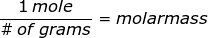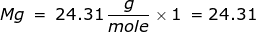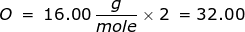# Grams to Moles Conversion Formula

Grams to Moles Conversion Formula

Many chemical equations require that the amount of substance be in the unit of moles. This leads to a very common conversion in which the grams of the substance be converted into moles.More commonly written in chemical conversions as:whereGrams to Moles Conversion Formula Questions:

1. How many moles are in 75.37 grams of sodium chloride, NaCl?

First, find the molar mass of NaCl. Use the periodic table to find the mass of each element then multiply that value by the number of each element's atoms in the particle. In this case, there is only one sodium (Na) atom and chlorine (Cl) atom in NaCl.Now use this value in the conversion.2. How many moles are in 122.5 grams of magnesium hydroxide, Mg(OH)2?